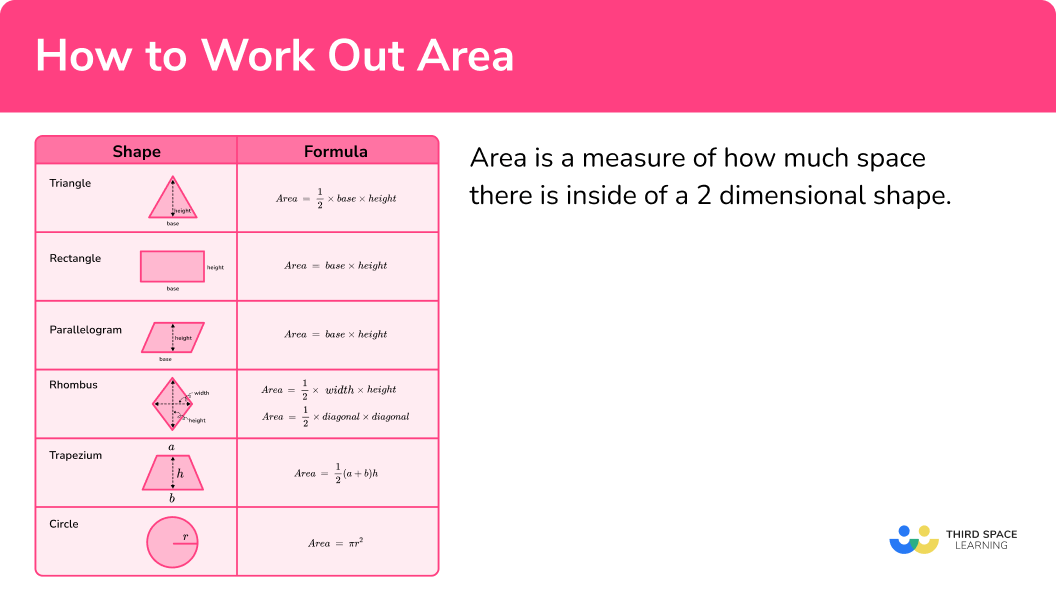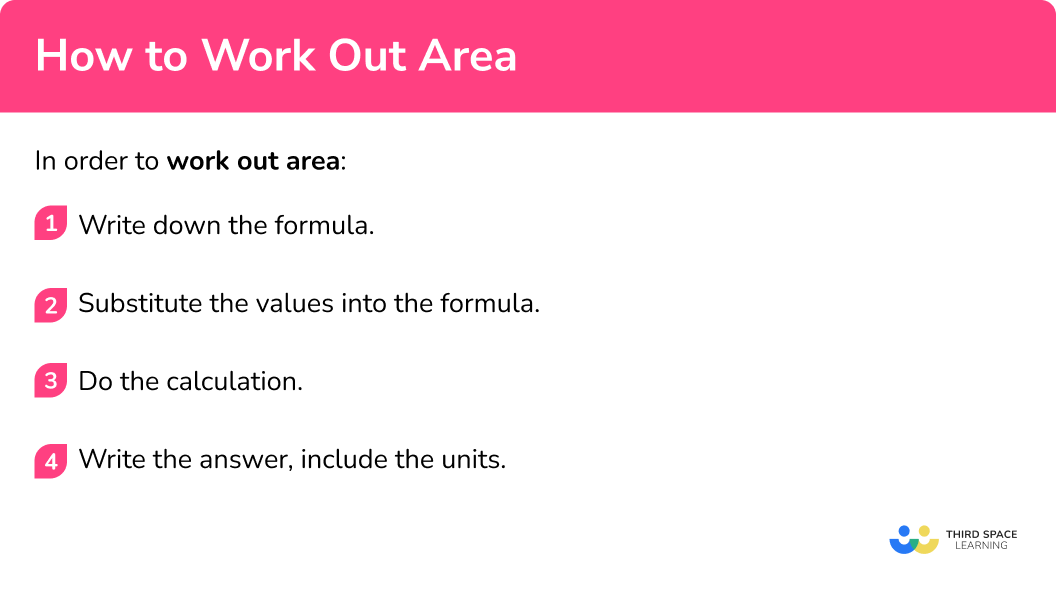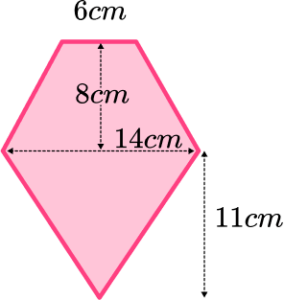GCSE Maths Geometry and Measure Area

How To Work Out Area

# How To Work Out Area

Here we will learn how to work out area.

There are also how to work out area worksheets based on Edexcel, AQA and OCR exam questions, along with further guidance on where to go next if you’re still stuck.

## What is area?

Area is a measure of how much space there is inside of a 2 dimensional shape. To find the area of a shape we can either count the number of unit squares within the shape or use the appropriate area formula for that shape.

Area is measured in square units e.g. cm^{2}, \; m^{2}, \; mm^{2} .

The table below shows the formulae for calculating area for some of the most common 2 D shapes:

### What is area?## How to work out area

In order to work out area:

1. Write down the formula.
2. Substitute the values into the formula.
3. Do the calculation.
4. Write the answer, including the units.

### How to work out area## Related lessons on area

How to work out area is part of our series of lessons to support revision on area. You may find it helpful to start with the main area lesson for a summary of what to expect, or use the step by step guides below for further detail on individual topics. Other lessons in this series include:

## How to work out area examples

### Example 1: area of a rectangle

Work out the area of the rectangle

1. Write down the formula.

\text{Area }= base \times height

2Substitute the values into the formula.

Here the base is 11cm and the height is 4cm.

Area=11 \times 4

3Do the calculation.

Area=11 \times 4

Area=44

4Write the answer, including the units.

The measurements are in centimetres so the area will be measured in square centimetres.

Area=44cm^2

### Example 2: area of a parallelogram

Work out the area of the parallelogram

Area= base \times height

Here the base is 10m and the height is 6m.

Area=10 \times 6

Area=10 \times 6

Area=60

The measurements are in metres so the area will be measured in square metres.

Area=60m^2

### Example 3: area of a rhombus

Calculate the area of the rhombus

\text{Area }=\frac{1}{2} \times \text{ width } \times \text{ height}

or \text{Area }= \frac{1}{2} \times \text{ diagonal } \times \text{ diagonal}

Here the width is 20mm and the height is 8mm.

\text{Area }=\frac{1}{2} \times 20 \times 8

\begin{aligned} \text{Area }&=\frac{1}{2} \times 20 \times 8\\\\ \text{Area }&=80 \end{aligned}

The measurements are in millimetres so the area will be measured in square millimetres.

Area=80mm^2

### Example 4: area of a trapezium

Find the area of the trapezium

\text{Area }=\frac{1}{2}(a+b)h

In a trapezium, a and b are the parallel sides and h is the height.

Here a=3, \; b=5 and h=4

\text{Area }=\frac{1}{2}(3+5) \times 4

\begin{aligned} &\text{Area }=\frac{1}{2}(3+5) \times 4\\\\ &\text{Area }=\frac{1}{2} \times 8 \times 4\\\\ &\text{Area }=16 \end{aligned}

The measurements are in cm so the area will be measured in cm^2 .

Area=16cm^2

### Example 5: area of a triangle

Work out the area of the triangle

\text{Area }=\frac{1}{2} \times \text{ base } \times \text{ height}

Since this is a right triangle, we can see the base of the triangle is 13mm and the height of the triangle is 6mm .

\text{Area }=\frac{1}{2} \times 13 \times 6

\begin{aligned} \text{Area }&=\frac{1}{2} \times 13 \times 6\\\\ \text{Area }&=39 \end{aligned}

The measurements are in mm so the area will be measured in mm^2 .

Area=39mm^2

### Example 6: area of a circle

Work out the area of the circle. Give your answer to 1 decimal place

\text{Area }=\pi r^{2}

Here the radius, r, is 3.5cm .

\text{Area }=\pi \times 3.5^{2}

\begin{aligned} \text{Area }&=\pi \times 3.5^{2}\\\\ \text{Area }&=38.48451001 \end{aligned}

We need to write the answer to one decimal place and include the units.

Area=38.5cm^2

## How to work out area of a compound shape

In order to work out area of a compound shape:

1. Draw lines to split the shape into two or more smaller shapes. Label the shapes A, B, C, …
2. Consider each shape individually.
a)  Work out any measurements that you need.
b) Calculate the area using the methods above.
3. Add or subtract the relevant areas to find the total area.
4. Write the answer, including the units.

## Area of a compound shape examples

### Example 7: area of a compound shape

Work out the area of the following shape

Shape A

a) Work out any measurements that you need.

For a rectangle, we need the height and the base,both of which we are given.

b) Calculate the area using the methods above.

\begin{aligned} \text{Area }&=\text{ base }\times \text{ height}\\\\ &=10 \times 6\\\\ &=60\mathrm{cm}^{2} \end{aligned}

Shape B

a) Work out any measurements that you need.

For a trapezium, we need a, \; b and h.

b) Calculate the area using the methods above.

\begin{aligned} \text{Area }&=\frac{1}{2}(a+b)h\\\\ &=\frac{1}{2}(5+10) \times 3\\\\ &=22.5\mathrm{cm}^{2} \end{aligned}

Total area: 60+22.5=82.5

\text{Area } =82.5cm^2

### Example 8: area of a compound shape

The shape is already clearly split.

Shape A

a) Work out any measurements that you need.

For a triangle we need the height and base, which we are given

b) Calculate the area using the methods above.

\begin{aligned} \text{Area }&= \frac{1}{2} \times \text{ base } \times \text{ height}\\\\ &=\frac{1}{2} \times 10 \times 14\\\\ &=70\mathrm{cm}^{2} \end{aligned}

Shape B

a) Work out any measurements that you need.

Here we need the radius of the circle, which is 3.2cm

b) Calculate the area using the methods above.

\begin{aligned} \text{Area }&=\pi \times r^{2}\\\\ &=\pi \times 3.2^{2}\\\\ &=32.16990877\\\\ &=32.17\mathrm{cm}^{2} \end{aligned}

\text{Area } =37.8cm^2

### Common misconceptions

• Using an incorrect formula

There are several different formulae for the different shapes – make sure you use the correct one.

• Using the incorrect units/not including units

Area is measured in square units.

E.g.

Square millimetres, square centimetres, square metres, square inches, square feet, square yards etc.

• Calculating with different units

All measurements must be in the same units before calculating surface area.

E.g.

You can’t have some measurements in cm and some in m.

• Calculating perimeter/circumference instead of area

Remember, perimeter is distance around the outside whilst area is the space inside the shape.

To work out the area of a circle we need the radius, which is the distance from the centre of the circle to the edge of the circle.

### Practice how to work out area questions

1. Work out the area of the square14\mathrm{cm}^{2}49\mathrm{cm}^{2}28\mathrm{cm}^{2}42\mathrm{cm}^{2}Since this is a square, the base and height are the same, 7cm.

\begin{aligned} \text{Area }&=\text{ base } \times \text{ height}\\\\ &=7 \times 7\\\\ &=49\mathrm{cm}^{2} \end{aligned}

2. Work out the area of the parallelogram55\mathrm{mm}^{2}18\mathrm{mm}^{2}36\mathrm{mm}^{2}77\mathrm{mm}^{2}The base is 11mm and the height is 5mm.

\begin{aligned} \text{Area }&=\text{ base } \times \text{ height }\\\\ &=11 \times 5\\\\ &=55\mathrm{mm}^{2} \end{aligned}

3. Calculate the area of the rhombus260\mathrm{m}^{2}33\mathrm{m}^{2}16.5\mathrm{m}^{2}130\mathrm{m}^{2}\begin{aligned} \text{Area }&=\frac{1}{2} \times \text{ width } \times \text{ height}\\\\ &=\frac{1}{2} \times 20 \times 13\\\\ &=130\mathrm{m}^{2} \end{aligned}

4. Find the area of the trapezium36\mathrm{cm}^{2}60\mathrm{cm}^{2}26\mathrm{cm}^{2}576\mathrm{cm}^{2}\begin{aligned} \text{Area }&=\frac{1}{2}(a+b)h\\\\ &=\frac{1}{2} (8+12) \times 6\\\\ &=60\mathrm{cm}^{2} \end{aligned}

5. Work out the area of the triangle36\mathrm{mm}^{2}13\mathrm{mm}^{2}18\mathrm{mm}^{2}72\mathrm{mm}^{2}\begin{aligned} \text{Area }&=\frac{1}{2} \times \text{ base } \times \text{ height}\\\\ &=\frac{1}{2} \times 9 \times 4\\\\ &=18\mathrm{mm}^{2} \end{aligned}

6. Calculate the area of the circle. Give your answer to 3sf.69.1\mathrm{m}^{2}1520\mathrm{m}^{2}34.6\mathrm{m}^{2}380\mathrm{m}^{2}\begin{aligned} \text{Area }&=\pi r^{2}\\\\ &=\pi \times 11^{2}\\\\ &=380.132711\\\\ &=380\mathrm{m}^{2} \end{aligned}

7. Work out the area of the compound shape418\mathrm{cm}^{2}550\mathrm{cm}^{2}561\mathrm{cm}^{2}286\mathrm{cm}^{2}Shape A:

\begin{aligned} \text{Area }&=\frac{1}{2} \times \text{ base } \times \text{ height}\\\\ &=\frac{1}{2} \times 22 \times 12\\\\ &=132\mathrm{cm}^{2} \end{aligned}

Shape B:

\begin{aligned} \text{Area }&=\text{ base } \times { height}\\\\ &=22 \times 13\\\\ &=286\mathrm{cm}^{2} \end{aligned}

Total area: 132+286=418cm^2

### How to work out area GCSE questions

1. Work out the area of this shape(3 marks)

\text{Area of trapezium: }\frac{1}{2}(6+14) \times 8=80\mathrm{cm}^{2}

(1)

\text{Area of triangle: }\frac{1}{2} \times 14 \times 11 = 77\mathrm{cm}^{2}

(1)

Total area: 80 + 77 = 157cm^2

(1)

2. Sean wants to paint both sides of a fence which is 20m long and 1.5m high.

One tin of the paint that Sean wants to use will cover 15m^2 . How many tins of paint should Sean buy?

(3 marks)

\text{Area of one side: } 20 \times 1.5 = 30\mathrm{m}^{2}

(1)

\text{Area of both sides: } 2 \times 30 = 60 \mathrm{m}^{2}

(1)

60 \div 15 = 4 tins

(1)

3. A shop sells pizza in two sizes: 10 inches and 13 inches. Would Louise get more pizza if she bought one large pizza or two small pizzas? Show how you decide.(3 marks)

\text{Small pizza: Area }=\pi \times 5^{2} = 78.54\text{ square inches}

\text{Two small pizzas: } 2\times 78.54=157.08 \text{ square inches}

(1)

\text{Large pizza: Area} = \pi \times 6.5^{2} = 132.72 \text{ square inches}

(1)

Two small pizzas

(1)

## Learning checklist

You have now learned how to:

• Work out the area of triangles, quadrilaterals and circles
• Work out the area of compound shapes

## Still stuck?

Prepare your KS4 students for maths GCSEs success with Third Space Learning. Weekly online one to one GCSE maths revision lessons delivered by expert maths tutors.

Find out more about our GCSE maths tuition programme.